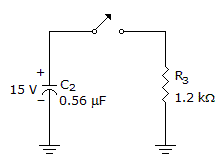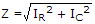# Electronics - Capacitors

### Exercise :: Capacitors - True or False

11.In the given circuit, the voltage across the capacitor 1 ms after the switch is closed is 11.61 V.

 A. True B. False

Explanation:

No answer description available for this question. Let us discuss.

12.

The frequency response curve illustrates that as the frequency of the input increases, the voltage of the output will decrease.

 A. True B. False

Explanation:

No answer description available for this question. Let us discuss.

13.

In a series capacitive circuit the smallest capacitor will have the largest voltage drop across it.

 A. True B. False

Explanation:

No answer description available for this question. Let us discuss.

14.

Capacitance is directly proportional to the dielectric constant and the distance between its plates and inversely proportional to the area of the plates.

 A. True B. False

Explanation:

No answer description available for this question. Let us discuss.

15.

The impedance in an RC parallel circuit isA. True B. False